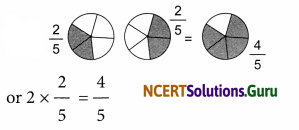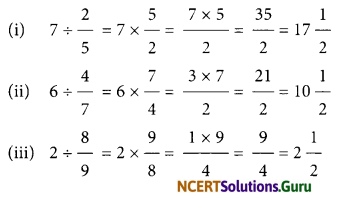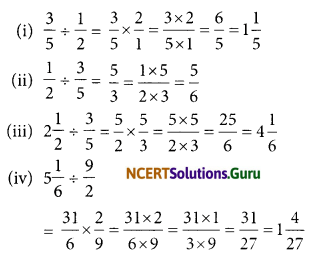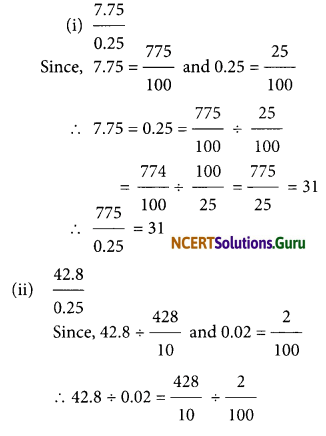# NCERT Solutions for Class 7 Maths Chapter 2 Fractions and Decimals InText Questions

These NCERT Solutions for Class 7 Maths Chapter 2 Fractions and Decimals InText Questions and Answers are prepared by our highly skilled subject experts.

## NCERT Solutions for Class 7 Maths Chapter 2 Fractions and Decimals InText Questions

NCERT In-text Question Page No. 34
Question 1.
Find:
(a) $$\frac { 1 }{ 2 }$$ × 3
(b) $$\frac { 9 }{ 7 }$$ × 6
(c) 3 × $$\frac { 1 }{ 8 }$$
(d) $$\frac { 13 }{ 11 }$$ × 6
if the product is an improper fraction express it as a mixed fractionQuestion 2.
Represent pictorially: 2 × $$\frac{2}{5}=\frac{4}{5}$$NCERT In-text Question Page No. 34
Question 1.
(i) 5 × 2 $$\frac{3}{7}$$
(ii) 1 $$\frac{4}{9}$$ × 6NCERT In-text Question Page No. 35
Question 1.
Can you tell, what is (i) $$\frac{1}{2}$$ of 10?
(i) $$\frac{1}{4}$$ of 16? (iii) (i) $$\frac{2}{5}$$ of 25?NCERT In-text Question Page No. 39
Question 1.
Fill in these boxes:NCERT In-text Question Page No. 40
Question 1.
Find:
$$\frac{1}{3} \times \frac{4}{5} ; \frac{2}{3} \times \frac{1}{5}$$Question 2.
Find:
$$\frac{8}{3} \times \frac{4}{7} \times \frac{3}{4} \times \frac{2}{3}$$NCERT In-text Question Page No. 44
Question 1.
(i) Will the reciprocal of a proper fraction be again a proper fraction?
(ii) Will the reciprocal of an improper fraction be again an improper fraction?
(i) No, the reciprocal of an improper fraction is a improper fraction.
(ii) No, the reciprocal of an improper fraction is a proper fraction.
Now, we can say that
(a) 1 ÷ $$\frac { 1 }{ 2 }$$ = 1 × $$\frac { 2 }{ 1 }$$ = 1 × reciprocal of $$\frac { 1 }{ 2 }$$

(b) 3 ÷ $$\frac { 1 }{ 4 }$$ = 3 × $$\frac { 4 }{ 1 }$$ = 3 × reciprocal of $$\frac { 1 }{ 4 }$$

(c) 3 ÷ $$\frac { 1 }{ 2 }$$ = ………….. = …………….. 3 ÷ $$\frac { 1 }{ 2 }$$ = 3 × $$\frac { 2 }{ 1 }$$ = 3 × reciprocal of $$\frac { 1 }{ 2 }$$
And, 2 ÷ $$\frac { 3 }{ 4 }$$ = 2 × reciprocal of $$\frac { 3 }{ 4 }$$ = 2 × $$\frac { 4 }{ 3 }$$

(d) 5 ÷ $$\frac { 2 }{ 9 }$$ = 5 × …………… = 5 × ……………..

∴ 5 ÷ $$\frac { 2 }{ 9 }$$ = 5 × $$\frac { 9 }{ 2 }$$ = 5 × reciprocal of $$\frac { 2 }{ 9 }$$

NCERT In-text Question Page No. 45
Question 1.
Find:
(i) 7 ÷ $$\frac { 2 }{ 5 }$$
(ii) 7 ÷ $$\frac { 4 }{ 7 }$$
(iii) 7 ÷ $$\frac { 8 }{ 9 }$$NCERT In-text Question Page No. 45
Question 1.
Find:
(i) 6 ÷ 5$$\frac { 1 }{ 3 }$$
(ii) 7 ÷ 2$$\frac { 4 }{ 7 }$$NCERT In-text Question Page No. 45
Question 1.
Find:
(i) $$\frac{3}{5} \div \frac{1}{2}$$
(ii) $$\frac{1}{2} \div \frac{3}{5}$$
(iii) 2$$\frac{1}{3} \div \frac{3}{5}$$
(iv) 5\frac{1}{6} \div \frac{9}{2}NCERT In-text Question Page No. 50
Question 1.
Find:
(i) 2.7 × 4
(ii) 1.8 × 1.2
(iii) 2.3 × 4.35
(i) 27 × 4 = 108 and there is one digit to the right of the decimal point in 27 2.7 × 4 = 10.8
(ii) 18 × 12 = 216 and number of digits to the right of decimal point is (1 + 1) 2 = 2
1.8 × 1.2 = 2.16

(iii) 23 × 435 = 10005 and there are 1 + 2 = 3 digits to the right of decimal point
2.3 × 4.35 = 10.005

What Is 5/8 As a Decimal? … The fraction five-eighths (5/8) is the decimal 0.625.

Question 2.
Arrange the products obtained in Question in descending order.
The products are 10.8, 2.16, 10.005. Comparing 10.8 and 10.005, we have :
10 = 10, 8 > 0, i.e. 10.005 < 10.8
Here, the smallest number = 2.16
and, the largest number = 10.8
Thus the required descending order is : 10.8, 10.005,2.16.NCERT In-text Question Page No. 51
Question 1.
Find:
(i) 0.3 × 10
(ii) 1.2 × 100
(iii) 56.3 × 1000
(i) 0.3 × 10
There is one zero in 10
∵ The decimal point is shifted to the right by one place
Thus, 0.3 × 10 = 3

(ii) There are 2 zeroes in 100
∵ The decimal point is shifted to the right by 2 places
= 1.20 × 100= 120
Thus, 1.2 × 100 = 120

(iii) There are 3 zeros in 1000
∵ The decimal point is shifted to the right by 3 places
Thus, 56.3 × 1000 = 56.300 × 1000 = 56300

In other words, we will show you how to write 11/16 as a decimal.

NCERT In-text Question Page No. 53
Question 1.
Find:
(i) 235.4 ÷ 10
(ii) 235.4 ÷ 100
(iii) 235.4 ÷ 1000
(i) 235.4 ÷ 10
Since, there is one zero in 10.
∴ The decimal point in the quotient is shifted to the left by one place.
∴ 235.4 ÷ 10 = 23.54

(ii) 235.4 ÷ 100
Since, there are two zeros in 100
∴ The decimal point in the quotient is
shifted to the left by three places.
∴ 235.4 H- 1000 = 0.2354NCERT In-text Question Page No. 53
Question 1.
Find:
(i) 35.7 ÷ 3 = ?
(ii) 25.5 ÷ 3 = ?
(i) 35.7 ÷ 3
Since, $$\frac{357}{3}$$ = 119 and there is one digit in the decimal part of the given decimal number.
∴ The decimal point is placed in the quotient after one digit from the right most digit.
∴ 35.7 ÷ 3 = 11.9

(ii) 25.5 ÷ 3
Since, 255 ÷ 3 = 85 and there is one digit in the decimal part of the given decimal number.
∴ The decimal is placed in the quotient after one digit from the right most digit.
∴ 25.5 ÷ 3 = 8.5

NCERT In-text Question Page No. 53

Question 1.
(i) 43.15 ÷ 5 = ?
(ii) 82.44 ÷ 6 = ?NCERT In-text Question Page No. 53
Question 1.
Find:
(i) 15.5 ÷ 5
(ii) 126.35 ÷ 7
(i) 15.5 ÷ 5
Since 155 ÷ 5 = 31 and there is one digit in the decimal part of the given decimal number.
∴ Place the decimal point in 31 such that there is one digit to its right.
∴ 15.5 + 5 = 3.1

(ii) 126.37 + 7
Since 12635 + 7 = 1805 and there are two digits in the decimal part of the given decimal number.
∴ Place the decimal point in 1805 such that there are two digits to its right.
∴ 126.35 ÷ 7 = 18.05NCERT In-text Question Page No. 54
Question 1.
Find:
(i) $$\frac{7.75}{0.25}$$
(ii) $$\frac{42.8}{0.02}$$
(iii) $$\frac{5.6}{1.4}$$